Published

# Hand Washing Timer That Sings to You!

This project uses an ultrasonic sensor to allow a "hand wave" (no touch!) to trigger a series of LED's and the Happy Birthday song twice

IntermediateFull instructions provided1,484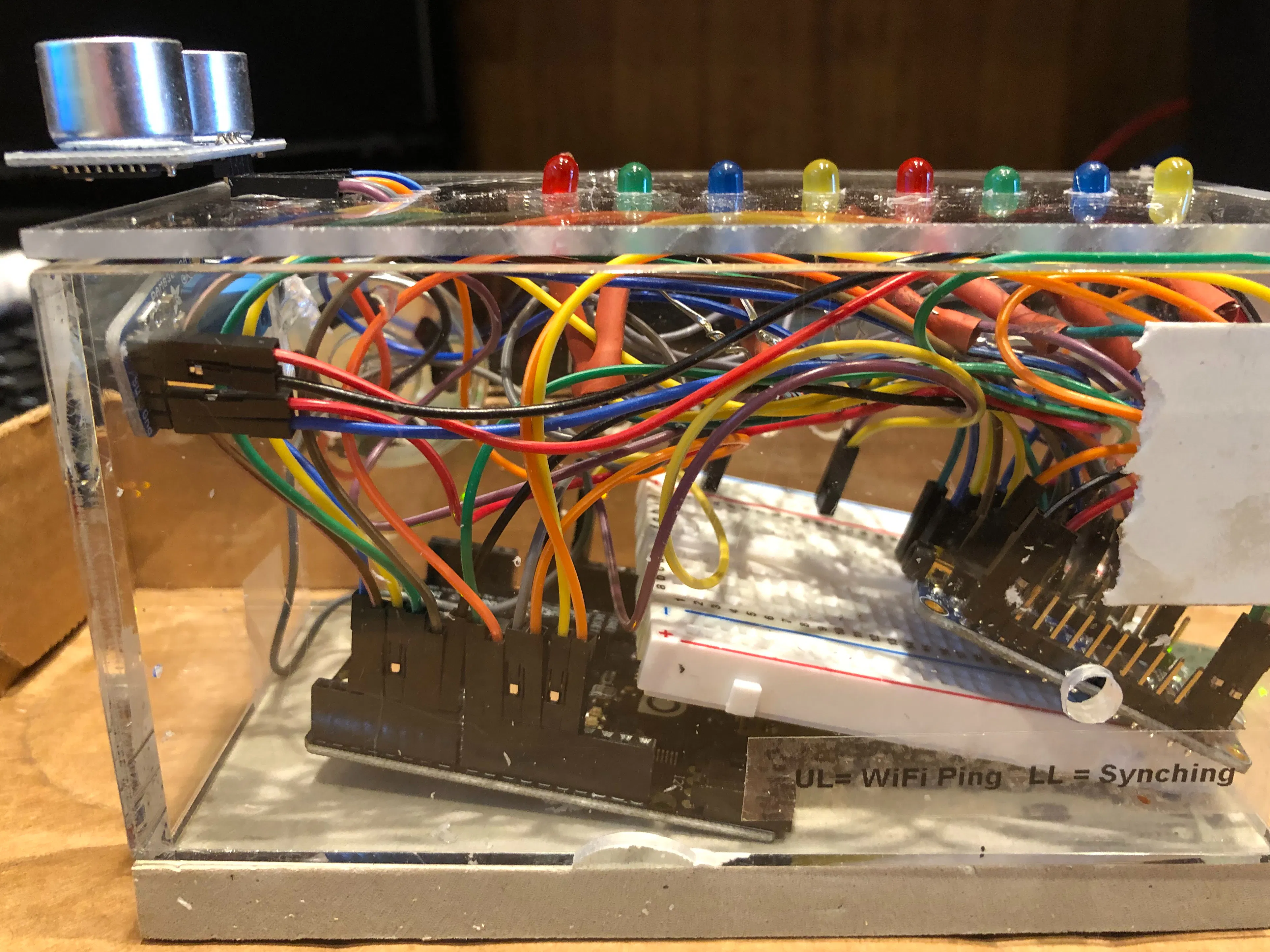## Things used in this project

### Hardware componentsArduino UNO
×1
 Adafruit FX Soundboard 2mb
×1Ultrasonic Sensor - HC-SR04 (Generic)
×1Breadboard (generic)
×1LED (generic)
×8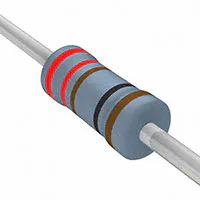Resistor 221 ohm
×8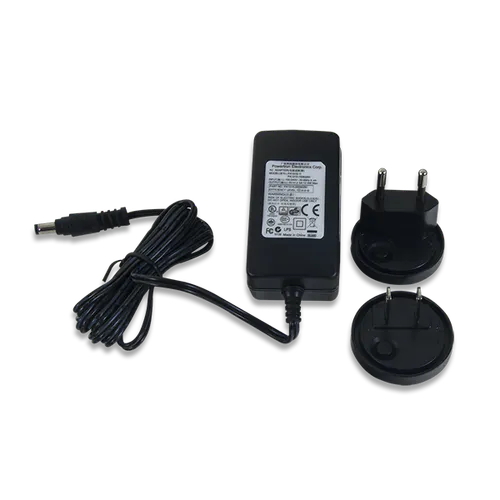Digilent 5V 2.5A Switching Power Supply
×1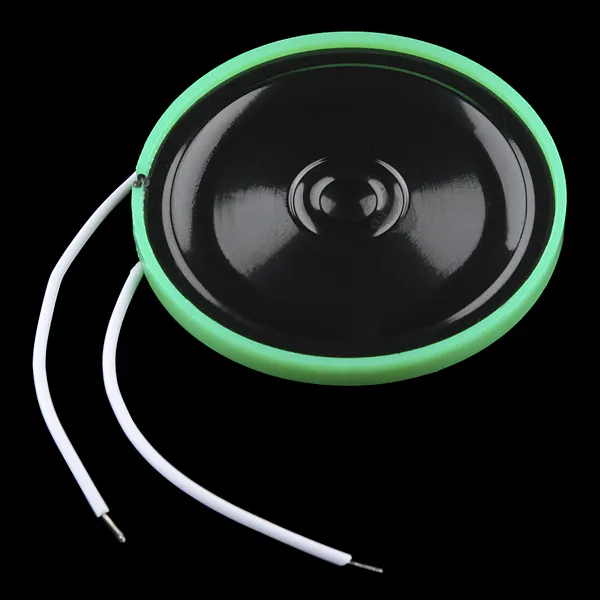Speaker: 0.25W, 8 ohms
×1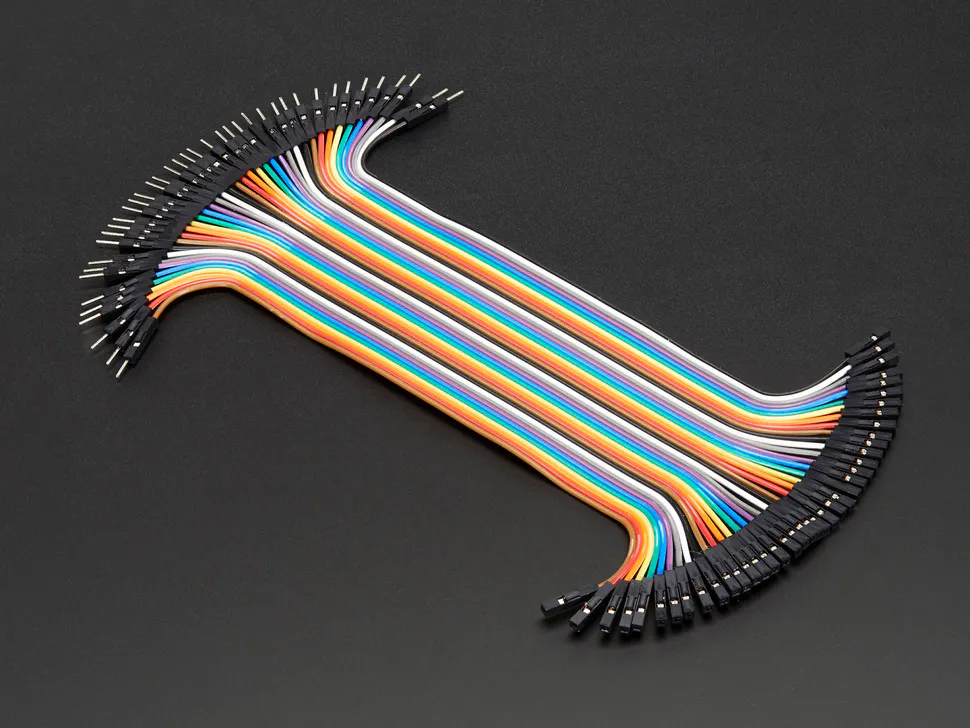Male/Female Jumper Wires
×1

Read more

## Code

### Arduino code

Arduino
Arduino IDE code
``` /* HC-SR04 example sketch
*
* https://create.arduino.cc/projecthub/Isaac100/getting-started-with-the-hc-sr04-ultrasonic-sensor-036380
*
* by Isaac100
Code

First we define the pins that Trig and Echo are connected to.

const int trigPin = 9;
const int echoPin = 10;
Then we declare 2 floats, duration and distance, which will hold the length of the sound wave and how far away the object is.

float duration, distance;
Next, in the setup, we declare the Trig pin as an output, the Echo pin as an input, and start Serial communications.

void setup() {
pinMode(trigPin, OUTPUT);
pinMode(echoPin, INPUT);
Serial.begin(9600);
}
Now, in the loop, what we do is first set the trigPin low for 2 microseconds just to make sure that the pin in low first. Then, we set it high for 10 microseconds, which sends out an 8 cycle sonic burst from the transmitter, which then bounces of an object and hits the receiver(Which is connected to the Echo Pin).

void loop() {
digitalWrite(trigPin, LOW);
delayMicroseconds(2);
digitalWrite(trigPin, HIGH);
delayMicroseconds(10);
digitalWrite(trigPin, LOW);
When the sound waves hit the receiver, it turns the Echo pin high for however long the waves were traveling for. To get that, we can use a handy Arduino function called pulseIn(). It takes 2 arguments, the pin you are listening to(In our case, the Echo pin), and a state(HIGH or LOW). What the function does is waits for the pin to go whichever state you put in, starts timing, and then stops timing when it switches to the other state. In our case we would put HIGH since we want to start timing when the Echo pin goes high. We will store the time in the duration variable. (It returns the time in microseconds)

duration = pulseIn(echoPin, HIGH);
Now that we have the time, we can use the equation speed = distance/time, but we will make it time x speed = distance because we have the speed. What speed do we have? The speed of sound, of course! The speed of sound is approximately 340 meters per second, but since the pulseIn() function returns the time in microseconds, we will need to have a speed in microseconds also, which is easy to get. A quick Google search for "speed of sound in centimeters per microsecond" will say that it is .0343 c/S. You could do the math, but searching it is easier. Anyway, with that information, we can calculate the distance! Just multiply the duration by .0343 and then divide it by 2(Because the sound waves travel to the object AND back). We will store that in the distance variable.

distance = (duration*.0343)/2;
The rest is just printing out the results to the Serial Monitor.

Serial.print("Distance: ");
Serial.println(distance);
delay(100);
}
*/

#define DDT                                 // define to turn on debugging tools
void DDTv(String st, int vt) {              // Print descriptor and value
#ifdef DDT
Serial.print("  ");
Serial.print(st);
Serial.print("  ");
Serial.print(vt);}
#endif
void DDTl(String st, int vt) {               // Print descriptor and value and new line
#ifdef DDT
DDTv(st, vt);
Serial.println(" ");}
#endif
void DDTs(String st) {                     // Print string
#ifdef DDT
Serial.print(st);}
#endif
void DDTt(String st) {                     // Print string and new line
#ifdef DDT
Serial.println(st);}
#endif
void DDTf(String st, float fi) {          // Print descriptor and floating point value and new line
#ifdef DDT
Serial.print("  ");
Serial.print(st);
Serial.print("  ");
Serial.println(fi,4);}
#endif
void DDTsl(String st, String vt) {                                                      // Print descriptor and string value and new line
#ifdef DDT
Serial.print(st);
Serial.println(vt);}
#endif

const int trigPin = 10;
const int echoPin = 11;
const int Wash_Time = 20;             // seconds to wash hands
const int LED_Qty = 8;                // number of LED_Pin
const int LED_Pin = 2;                // first LED pin
const int soundpin = 12;                // trigger for song
const int actpin = 13;                  // will be "HIGH" while playing
const int Trigger_Distance = 50;     // closeness to trigger a wash

float duration, distance;

void setup() {
pinMode(trigPin, OUTPUT);
pinMode(echoPin, INPUT);
pinMode(actpin, INPUT);
pinMode(soundpin, OUTPUT);
digitalWrite(soundpin,HIGH);
for (int i = 0; i < LED_Qty; i++) {digitalWrite (LED_Pin+i, LOW);}

Serial.begin(9600);

}   // end of setup

void loop() {
digitalWrite(trigPin, LOW);
delayMicroseconds(2);
digitalWrite(trigPin, HIGH);
delayMicroseconds(10);
digitalWrite(trigPin, LOW);

duration = pulseIn(echoPin, HIGH);
distance = (duration*.0343)/2;                                    // convert using speed of sound to centimeters
Serial.print("Distance: ");
Serial.println(distance);
if (distance <= Trigger_Distance) {Wash_Them();}
delay(100);
} // end of loop

void Wash_Them() {
DDTt("Wash Them Called");
Flash(3);                                                       // flash for two seconds
digitalWrite(soundpin,LOW);                                    //trigger the song
delay(150);                                                     //hold just a bit then
digitalWrite(soundpin,HIGH);                                     // and turn it off (it will play through)
do {
for (int i = 0; i < LED_Qty; i++) {
digitalWrite (LED_Pin+i, HIGH);
delay(400);
digitalWrite (LED_Pin+i, LOW);
}  // LED loop
DDTl("Active pin is ",digitalRead(actpin));
}   while (digitalRead(actpin) == LOW);                          // play till the song is done
Flash(4);                                                       // flash again for two seconds

}  // end of Wash_Them

void Flash(int flashtime){
int ftimer = flashtime;
for (int ii = 0; ii< flashtime; ii++){
for (int i = 0; i < LED_Qty; i++) {
digitalWrite (LED_Pin+i, HIGH);}
delay(500);
for (int i = 0; i < LED_Qty; i++) {
digitalWrite (LED_Pin+i, LOW); }
delay(500);
}
}  // end of flash
```

## Credits

### spudnut1

7 projects • 11 followers